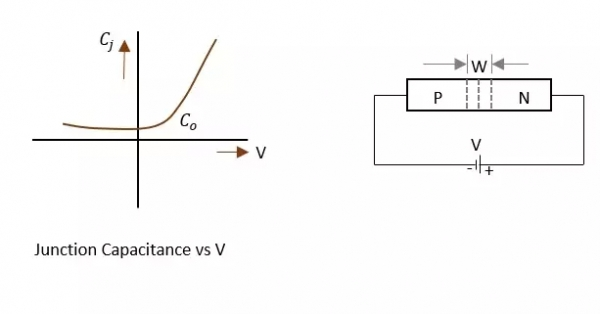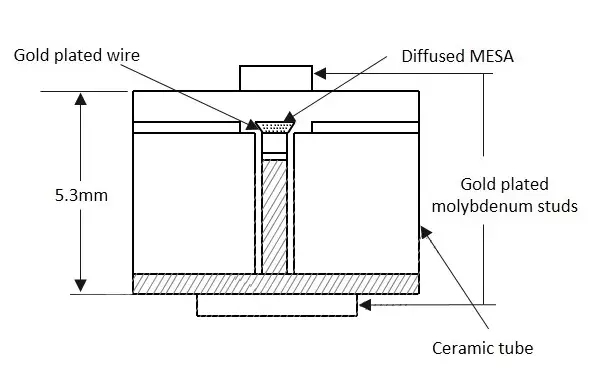# Explain the construction and working of a Microwave Varactor diode.

A voltage variable capacitance of a reverse biased junction can be termed as a Varactor diode. A varactor diode is a semiconductor device in which the junction capacitance can be varied as a function of the reverse bias of the diode.

The CV characteristics of a typical Varactor diode and its symbols are shown in the below figure.The junction capacitance depends on the applied voltage and junction design. If the junction is reverse biased, the mobile carriers deplete the junction, resulting in some capacitance, where the diode behaves as a capacitor, with junction acting as a dielectric. The capacitance decreases with the increase in reverse bias.

## Construction

The encapsulation of the diode contains electrical leads which are attached to the semiconductor wafer and a lead attached to the ceramic case. The below figure shows how a microwave Varactor diode looks.These are capable of handling large powers and large reverse breakdown voltages. These have low noise. Although variation in junction capacitance is an important factor in this diode, parasitic resistances, capacitances and conductances are associated with every practical diode, which should be kept low.

## Applications

There are many applications of Varactor diode such as:

• Harmonic generation
• Upconversion
• Parametric amplifier
• Pulse generation
• Pulse shaping
• Used in switching circuits
• Modulation of microwave signals.

Updated on: 30-Jul-2019

579 Views